#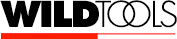Bisector Line Tool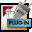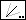Bisector Line Tool The Bisector Line tool allows you to divide an angle formed by two lines or the sides of any rectangle or polygon, even if in a group.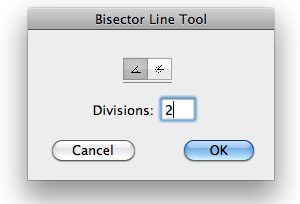In the tool's dialog, choose between dividing the inside or outside angle. Turn on the inside angle choice if you want to divide the acute angle formed by two lines. Select the outside angle if you want to divide the obtuse angle formed by the two lines. Type the number of divisions to divide the angle.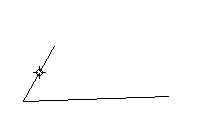To use the tool, place the cursor in the drawing area on the first object and press the mouse button. Drag to the second object and release the mouse button. New lines will be placed in the drawing to divide the angle. Note that the lines begin at the intersection of the two original lines and that the lengths of the lines are evenly stepped for a fan effect that may be useful at times. The way this angle-dividing process works is this: The intersection point is calculated, then the Bisector Line tool finds the end point of each line which is the farthest away from the intersection point, and these three points are used to calculate the angle. If you remember this, you will always be able to predict which angle will be divided when the two lines cross each other.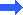Go back to WildTools